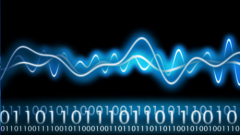## Digital Signal Processing

 所在平台: Coursera 课程类别: 工程Explore 1600+ online courses from top universities. Join Coursera today to learn data science, programming, business strategy, and more.#### 课程详情

The goal of the course is to develop a complete working set of digital signal processing notions from the ground up. DSP is arguably at the heart of the “digital revolution” that, in the space of just a few decades, has enabled unprecedented levels of interpersonal communication and of information availability.

In the class, starting from the basic definitions of a discrete-time signal, we will work our way through Fourier analysis, filter design, sampling, interpolation and quantization to build a DSP toolset complete enough to analyze a practical communication system in detail. Hands-on examples and demonstration will be routinely used to close the gap between theory and practice.

#### 课程大纲

Broad outline of the topics covered in the class:

1. Introduction: What is signal processing, history of the topic, application examples.
2. Discrete-time (DT) signals: the discrete-time complex exponential, and a computer music synthesis example.
3. Euclid and Hilbert: Signal processing as geometry, vectors spaces, bases, approximations.
4. Fourier Analysis: The discrete Fourier transform (DFT) and series (DFS). The discrete-time Fourier transform (DTFT). Examples. The fast Fourier transform algorithm (FFT).
5. Linear Filters: Linear time-invariant systems, convolution, ideal and realizable filters. Filter design and implementation, examples.
6. Interpolation and Sampling: Continuous-time (CT) signals, interpolation, sampling. The sampling theorem as orthonormal basis expansion. Processing of CT signals in DT.
7. Stochastic Signal Processing and Quantization: Stochastic signals, quantization, analog-to-digital (ADC) and digital-to-analog (DAC) conversion.
8. Image Processing: Introduction to image processing and two-dimensional (2D) Fourier analysis. Filtering and compression. The JPEG compression standard.
9. Digital Communication Systems: Analog channels and bandwidth/power constraints. Modulation and demodulation. Transmitter and receiver design. ADSL.